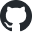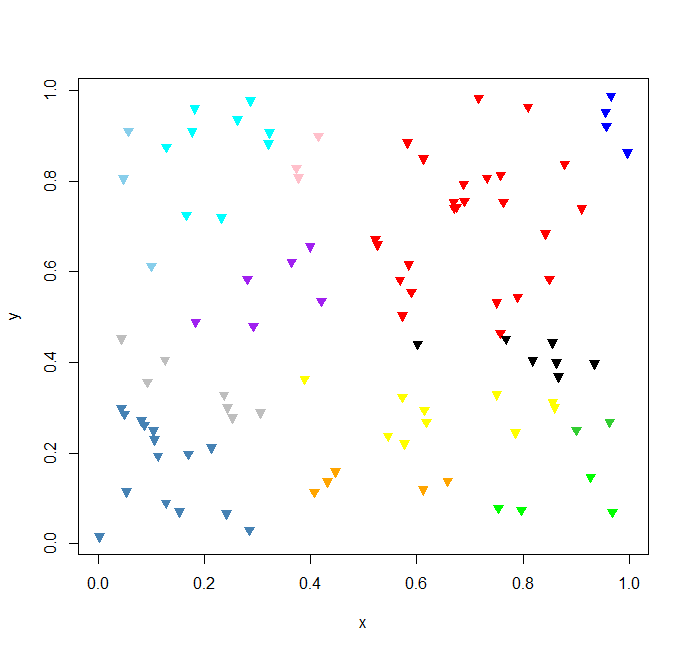##https://github.com/xieguigang/bclusterTree## 二叉树聚类的原理

1. 假若这两个键值的相似度比较低，例如，相似度小于0.6，那我们就给比较结果返回一个-1
2. 假若两个键值的相似度非常高，高过了我们所设定的一个阈值，例如相似度大于0.95，以至于我们可以近似的认为二者相等，那这个时候我们就给比较结果返回零
3. 假若相似度计算结果处于上面的两个情形之间，我们就返回1

## 二叉树聚类的应用https://github.com/SMRUCC/GCModeller/blob/master/images/phenotypic-bTree-lightbox.png

## 使用R语言实现二叉树聚类

1. 我们首先定义一个聚类的索引函数，
2. 然后将索引函数和等待聚类的entity序列一块传递到bcluster函数之中
3. 然后就可以得到聚类结果了，聚类结果是以索引号列表的形式返回的

# raw data
x   = runif(100);
y   = runif(100);
seq = lapply(1:length(x), function(i) list(v = c(x[i], y[i])));

# define compares order
index = function(p1, p2) {
d = sqrt(sum((p1$v - p2$v) ^ 2));

if (d <= 0.3) {
0
} else if (d <= 0.6) {
1
} else {
-1
}
}

# run clustering
c = bcluster(seq, index);

# plot result data
plot(x, y, pch = 2, col = "white");

i       = 1;
colors1 = c(
"red", "blue", "green", "yellow", "steelblue",
"purple", "black", "orange", "gray", "cyan",
"pink", "skyblue", "limegreen"
);

for(name in names(c)) {
points(x[c[[name]]], y[c[[name]]], pch=25, col=colors1[i], bg=colors1[i]);
i = i + 1;
}